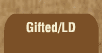Mathematics and Dyslexia

Tips for Learning the Times Tables

Tools for the Times Tables & Workbook

Home > Math > times tables practice > Sevens (big, scrambled)

## The sevens Times Tables - second set

Practice this page until they are fast and easy. This page should be as fast and easy as the 5s or the 1s. If you're having to stop and think a lot here, it won't get easier when these are mixed up with other numbers!

1. 7 x 7=
2. 7 x 10=
3. 7 x 6=
4. 8 x 7=
5. 6 x 7=
6. 7 x 9=
7. 7 x 8=
8. 7 x 7=
9. 9 x 7=
10. 8 x 7=
11. 6 x 7=
12. 7 x 9=
13. 7 x 7=
14. 7 x 10=
15. 8 x 7=